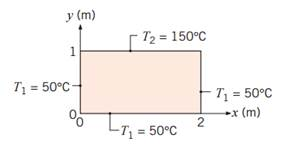### Create an Account

Already have account?

### Forgot Your Password ?

Home / Questions / Consider the two dimensional rectangular plate of Problem 42 having a thermal conductivity...

# Consider the two dimensional rectangular plate of Problem 42 having a thermal conductivity of 50 W m K Beginning with the exact solution for the temperature distribution derive an expression for

Consider the two-dimensional rectangular plate of Problem 4.2 having a thermal conductivity of 50 W/m∙ K. Beginning with the exact solution for the temperature distribution, derive an expression for the heat transfer rate per unit thickness from the plate along the lower surface (0 ≤ x ≤ 2, y = 0). Evaluate the heat rate considering the first five nonzero terms of the infinite series.

Problem 4.2

A two-dimensional rectangular plate is subjected to prescribed boundary conditions. Using the results of the exact solution for the heat equation presented in Section 4.2, calculate the temperature at the midpoint (1, 0.5) by considering the first five nonzero terms of the infinite series that must be evaluated. Assess the error resulting from using only the first three terms of the infinite series. Plot the temperature distributions T(x, 0.5) and T(1.0, y).View less »

Jun 28 2020 View more View Less

#### Answer (Solved)Subscribe To Get Solution